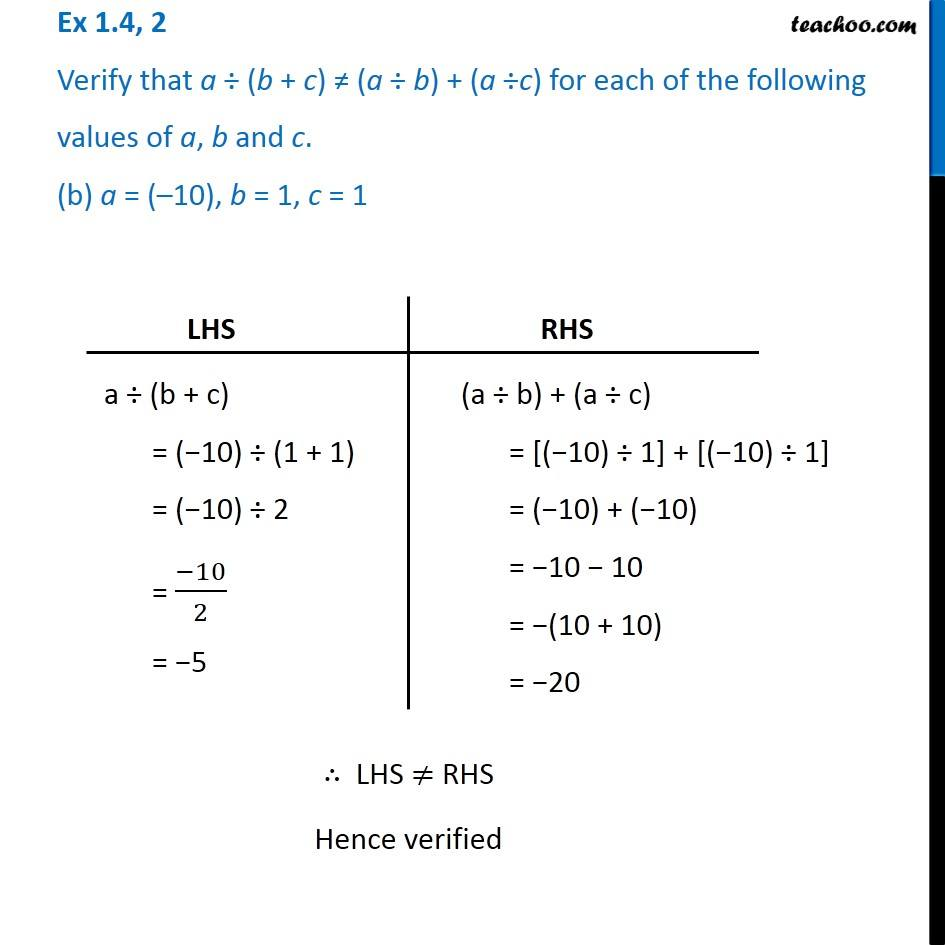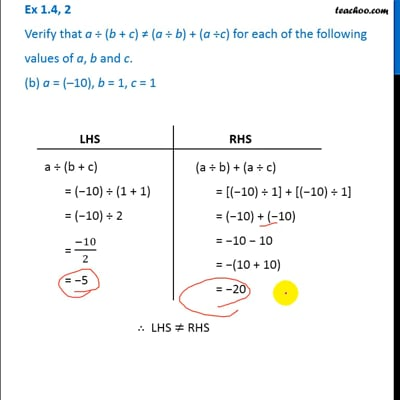Ex 1.4

Chapter 1 Class 7 Integers
Serial order wiseThis video is only available for Teachoo black users

### Transcript

Ex 1.4, 2 Verify that a (b + c) (a b) + (a c) for each of the following values of a, b and c. (b) a = ( 10), b = 1, c = 1 LHS a (b + c) = ( 10) (1 + 1) = ( 10) 2 = ( 10)/2 = 5 RHS (a b) + (a c) = [( 10) 1] + [( 10) 1] = ( 10) + ( 10) = 10 10 = (10 + 10) = 20 LHS RHS Hence verified